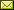## Characterizing Polynomial Time Computability of Rational and Real Functions

 Walid Gomaa (Alexandria University)

 Recursive analysis was introduced by A. Turing , A. Grzegorczyk , and D. Lacombe . It is based on a discrete mechanical framework that can be used to model computation over the real numbers. In this context the computational complexity of real functions defined over compact domains has been extensively studied. However, much less have been done for other kinds of real functions. This article is divided into two main parts. The first part investigates polynomial time computability of rational functions and the role of continuity in such computation. On the one hand this is interesting for its own sake. On the other hand it provides insights into polynomial time computability of real functions for the latter, in the sense of recursive analysis, is modeled as approximations of rational computations. The main conclusion of this part is that continuity does not play any role in the efficiency of computing rational functions. The second part defines polynomial time computability of arbitrary real functions, characterizes it, and compares it with the corresponding notion over rational functions. Assuming continuity, the main conclusion is that there is a conceptual difference between polynomial time computation over the rationals and the reals manifested by the fact that there are polynomial time computable rational functions whose extensions to the reals are not polynomial time computable and vice versa.

In S. Barry Cooper and Vincent Danos: Proceedings Fifth Workshop on Developments in Computational Models — Computational Models From Nature (DCM 2009), Rhodes, Greece, 11th July 2009, Electronic Proceedings in Theoretical Computer Science 9, pp. 54–64.
Published: 15th November 2009.

 ArXived at: http://dx.doi.org/10.4204/EPTCS.9.7 bibtex PDF

 Comments and questions to:eptcs@eptcs.org For website issues:webmaster@eptcs.org